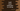# How to insert characters to a string in Swift## How to insert characters to a string in Swift:

In this post, we will learn how to insert characters to a string in swift. Swift string provides a couple of methods to add single or multiple characters to a string. In this post, we will learn how to use these methods with examples.

### Insert a single character using insert:

insert is an instance method of the String class. This is defined as below:

``mutating func insert(_ newElement: Character, at i: String.Index)``

Here, newElement - This is the character that we want to insert to the string. i - This is the index in the string where we want to insert the character. If it is equal to the end index of the string, it will append the character to the string. For invalid index, it will throw one Fatal error that string index is out of bounds

#### Example:

Let’s take a look at the below example:

``````var givenString = "Hello World"
let fourthIndex = givenString.index(givenString.startIndex, offsetBy: 4)

givenString.insert("x", at: fourthIndex)

print(givenString)``````

In this example, we are inserting the character x after the fourth character. It will print:

``Hellxo World``

Similarly, we can add characters to the start and end of a string:

``````var givenString = "Hello World"

givenString.insert("(", at: givenString.startIndex)
givenString.insert(")", at: givenString.endIndex)

print(givenString)``````

It prints :

``(Hello World)``

### Insert multiple characters using insert(contentsOf:at:):

insert(contentsOf:at:) is an instance method. This is defined as below :

``func insert<S>(contentsOf: S, at: String.Index)``

We can pass one string to insert at a specific Index. For example:

``````var givenString = "Hello World"

givenString.insert(contentsOf: "***", at: givenString.startIndex)
givenString.insert(contentsOf: "***", at: givenString.endIndex)

print(givenString)``````

It will print:

``***Hello World***``